Example problem: Unsteady flow in a 2D channel, driven by an applied traction

In this example we consider a variation of the unsteady 2D channel flow problem considered elsewhere. In the previous example the flow was driven by the imposed wall motion. Here we shall consider the case in which the flow is driven by an applied traction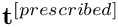which balances the fluid stress so that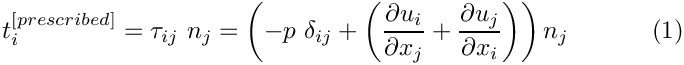along the upper, horizontal boundary of the channel. Here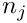is the outward unit normal,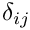is the Kronecker delta and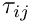the stress tensor. oomph-lib provides traction elements that can be applied along a domain boundary to (weakly) impose the above boundary condition. The traction elements are used in the same way as flux-elements in the Poisson and unsteady heat examples. The section Comments and Exercises at the end of this documents provides more detail on the underlying theory and its implementation in oomph-lib.

# The example problem

We consider the unsteady finite-Reynolds-number flow in a 2D channel that is driven by an applied traction along its upper boundary.

 Unsteady flow in a 2D channel, driven by an applied traction. Here is a sketch of the problem: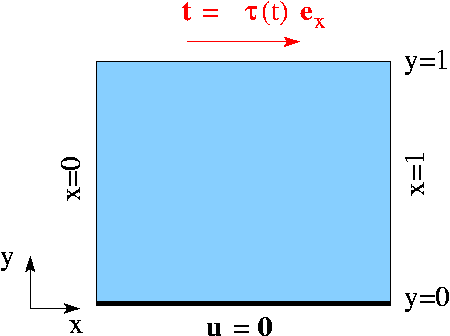Sketch of the problem. The flow is governed by the 2D unsteady Navier-Stokes equations,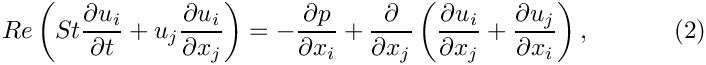and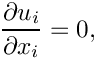in the square domain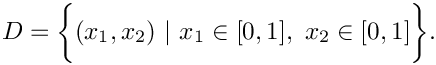We apply the Dirichlet (no-slip) boundary condition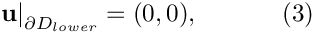on the lower, stationary wall,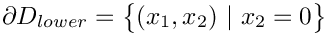and the traction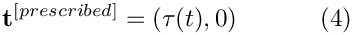where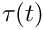is a given function, on the upper boundary,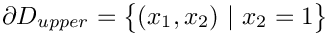. As in the previous example we apply periodic boundary conditions on the "left" and "right" boundaries: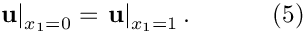Initial conditions for the velocities are given by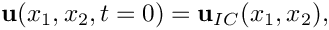where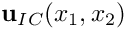is given.

## An exact, parallel-flow solution

We choose the prescribed traction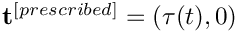such that the parallel-flow solution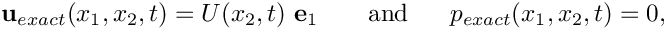derived in the previous example remains valid. For this purpose we set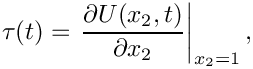where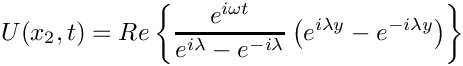and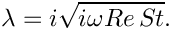# Results

The two animations below show the computed solutions obtained from a spatial discretisation with Taylor-Hood and Crouzeix-Raviart elements, respectively. In both cases we set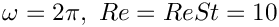and specified the exact, time-periodic solution as the initial condition, i.e.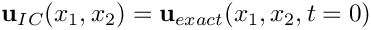. The computed solutions agree extremely well with the exact solution throughout the simulation.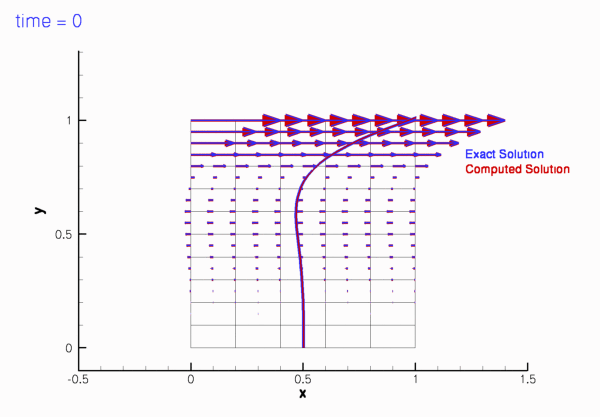Plot of the velocity field computed with 2D Crouzeix-Raviart elements, starting from the exact, time-periodic solution.Plot of the velocity field computed with 2D Taylor-Hood elements, starting from the exact, time-periodic solution.

# The global parameters

As usual, we use a namespace to define the problem parameters, the Reynolds number,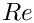, and the Womersley number,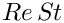. We also provide two flags that indicate the length of the run (to allow a short run to be performed when the code is run as a self-test), and the initial condition (allowing a start from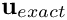or an impulsive start in which the fluid is initially at rest).

//===start_of_namespace=================================================
/// Namepspace for global parameters
//======================================================================
{
/// Reynolds number
double Re;
/// Womersley = Reynolds times Strouhal
double ReSt;
/// Flag for long/short run: Default = perform long run
unsigned Long_run_flag=1;
/// Flag for impulsive start: Default = start from exact
/// time-periodic solution.
} // end of namespace
Namepspace for global parameters.
unsigned Long_run_flag
Flag for long/short run: Default = perform long run.
double ReSt
Womersley = Reynolds times Strouhal.
double Re
Reynolds number.
unsigned Impulsive_start_flag
Flag for impulsive start: Default = start from exact time-periodic solution.

# The exact solution

We use a second namespace to define the time-periodic, parallel flow, and the tractionrequired to makea solution of the problem.

//==start_of_exact_solution=============================================
/// Namespace for exact solution
//======================================================================
namespace ExactSoln
{
/// Exact solution of the problem as a vector
void get_exact_u(const double& t, const Vector<double>& x, Vector<double>& u)
{
double y=x;
// I=sqrt(-1)
complex<double> I(0.0,1.0);
// omega
double omega=2.0*MathematicalConstants::Pi;
// lambda
complex<double> lambda(0.0,omega*Global_Parameters::ReSt);
lambda = I*sqrt(lambda);
// Solution
complex<double> sol(
exp(complex<double>(0.0,omega*t)) *
(exp(lambda*complex<double>(0.0,y))-exp(lambda*complex<double>(0.0,-y)))
/(exp(I*lambda)-exp(-I*lambda)) );
// Extract real part and stick into vector
u.resize(2);
u=real(sol);
u=0.0;
}
/// Traction required at the top boundary
void prescribed_traction(const double& t,
const Vector<double>& x,
const Vector<double> &n,
Vector<double>& traction)
{
double y=x;
// I=sqrt(-1)
complex<double> I(0.0,1.0);
// omega
double omega=2.0*MathematicalConstants::Pi;
// lambda
complex<double> lambda(0.0,omega*Global_Parameters::ReSt);
lambda = I*sqrt(lambda);
// Solution
complex<double> sol(
exp(complex<double>(0.0,omega*t)) *
(exp(lambda*complex<double>(0.0,y))+exp(lambda*complex<double>(0.0,-y)))
*I*lambda/(exp(I*lambda)-exp(-I*lambda)) );
//Extract real part and stick into vector
traction.resize(2);
traction=real(sol);
traction=0.0;
}
} // end of exact_solution
Namespace for exact solution.
void get_exact_u(const double &t, const Vector< double > &x, Vector< double > &u)
Exact solution of the problem as a vector.
void prescribed_traction(const double &t, const Vector< double > &x, const Vector< double > &n, Vector< double > &traction)
Traction required at the top boundary.

# The driver code

As in the previous example we use optional command line arguments to specify which mode the code is run in: Either as a short or a long run (indicated by the first command line argument being 0 or 1, respectively), and with initial conditions corresponding to an impulsive start or a start from the time-periodic exact solution (indicated by the second command line argument being 1 or 0, respectively). If no command line arguments are specified the code is run in the default mode, specified by parameter values assigned in the namespace Global_Parameters.

//===start_of_main======================================================
/// Driver code for Rayleigh-type problem
//======================================================================
int main(int argc, char* argv[])
{
/// Convert command line arguments (if any) into flags:
if (argc==1)
{
cout << "No command line arguments specified -- using defaults." << std::endl;
}
else if (argc==3)
{
cout << "Two command line arguments specified:" << std::endl;
// Flag for long run
/// Flag for impulsive start
}
else
{
std::string error_message =
"Wrong number of command line arguments. Specify none or two.\n";
error_message +=
"Arg1: Long_run_flag [0/1]\n";
error_message +=
"Arg2: Impulsive_start_flag [0/1]\n";
throw OomphLibError(error_message,
OOMPH_CURRENT_FUNCTION,
OOMPH_EXCEPTION_LOCATION);
}
cout << "Long run flag: "
cout << "Impulsive start flag: "
int main(int argc, char *argv[])
Driver code for Rayleigh-type problem.

Next we set the physical and mesh parameters.

// Set physical parameters:
// Womersly number = Reynolds number (St = 1)
//Horizontal length of domain
double lx = 1.0;
//Vertical length of domain
double ly = 1.0;
// Number of elements in x-direction
unsigned nx=5;
// Number of elements in y-direction
unsigned ny=10;

Finally we set up DocInfo objects and solve for both Taylor-Hood elements and Crouzeix-Raviart elements.

// Solve with Crouzeix-Raviart elements
{
// Set up doc info
DocInfo doc_info;
doc_info.number()=0;
doc_info.set_directory("RESLT_CR");
//Set up problem
problem(nx,ny,lx,ly);
}
// Solve with Taylor-Hood elements
{
// Set up doc info
DocInfo doc_info;
doc_info.number()=0;
doc_info.set_directory("RESLT_TH");
//Set up problem
problem(nx,ny,lx,ly);
}
} // end of main
Rayleigh-type problem: 2D channel flow driven by traction bc.

# The problem class

The problem class remains similar to that in the previous example. Since we are no longer driving the flow by prescribing a time-periodic tangential velocity at the upper wall, the function actions_before_implicit_timestep() can remain empty.

//===start_of_problem_class=============================================
/// Rayleigh-type problem: 2D channel flow driven by traction bc
//======================================================================
template<class ELEMENT, class TIMESTEPPER>
class RayleighTractionProblem : public Problem
{
public:
/// Constructor: Pass number of elements in x and y directions and
/// lengths
RayleighTractionProblem(const unsigned &nx, const unsigned &ny,
const double &lx, const double &ly);
/// Update before solve is empty
/// Update after solve is empty
/// Actions before timestep (empty)
/// Doc the solution
void doc_solution(DocInfo& doc_info);
/// Set initial condition (incl previous timesteps) according
/// to specified function.
void doc_solution(DocInfo &doc_info)
Doc the solution.
RayleighTractionProblem(const unsigned &nx, const unsigned &ny, const double &lx, const double &ly)
Constructor: Pass number of elements in x and y directions and lengths.
void set_initial_condition()
Set initial condition (incl previous timesteps) according to specified function.
void actions_after_newton_solve()
Update after solve is empty.
void actions_before_newton_solve()
Update before solve is empty.
void actions_before_implicit_timestep()
Actions before timestep (empty)

The function create_traction_elements(...) (discussed in more detail below) creates the traction elements and "attaches" them to the specified boundary of the "bulk" mesh.

/// Create traction elements on boundary b of the Mesh pointed
/// to by bulk_mesh_pt and add them to the Mesh object pointed to by
/// surface_mesh_pt
void create_traction_elements(const unsigned &b,
Mesh* const &bulk_mesh_pt,
Mesh* const &surface_mesh_pt);

The traction boundary condition sets the pressure so the function fix_pressure(...) used in the previous example is no longer required. The problem's private member data contains pointers to the bulk and surface meshes and the output stream that we use to record the time-trace of the solution.

private:
/// Pointer to the "bulk" mesh
/// Pointer to the "surface" mesh
Mesh* Surface_mesh_pt;
/// Trace file
ofstream Trace_file;
}; // end of problem_class

# The problem constructor

We start by building the timestepper, determining its type from the class's second template argument, and pass a pointer to it to the Problem, using the function Problem::add_time_stepper_pt(...).

//===start_of_constructor=============================================
/// Problem constructor
//====================================================================
template<class ELEMENT,class TIMESTEPPER>
(const unsigned &nx, const unsigned &ny,
const double &lx, const double& ly)
{
//Allocate the timestepper

Next we build the periodic bulk mesh,

//Now create the mesh with periodic boundary conditions in x direction
bool periodic_in_x=true;
Bulk_mesh_pt =
time_stepper_pt());

and the surface mesh,

// Create "surface mesh" that will contain only the prescribed-traction
// elements. The constructor just creates the mesh without
// giving it any elements, nodes, etc.
Surface_mesh_pt = new Mesh;

and use the function create_traction_elements(...) to populate it with traction elements that attach themselves to the specified boundary (2) of the bulk mesh.

// Create prescribed-traction elements from all elements that are
// adjacent to boundary 2, but add them to a separate mesh.
create_traction_elements(2,Bulk_mesh_pt,Surface_mesh_pt);

We add both sub-meshes to the Problem, using the function Problem::add_sub_mesh(...) and use the function Problem::build_global_mesh() to combine the sub-meshes into the Problem's single, global mesh.

// Add the two sub meshes to the problem
// Combine all submeshes into a single Mesh
build_global_mesh();

We apply Dirichlet boundary conditions where required: No-slip on the stationary, lower wall, at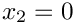, parallel outflow on the left and right boundaries, at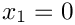and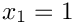. No velocity boundary conditions are applied at the "upper" boundary, at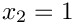, where the traction boundary condition is applied.

// Set the boundary conditions for this problem: All nodes are
// free by default -- just pin the ones that have Dirichlet conditions
// here
unsigned num_bound=Bulk_mesh_pt->nboundary();
for(unsigned ibound=0;ibound<num_bound;ibound++)
{
unsigned num_nod=Bulk_mesh_pt->nboundary_node(ibound);
for (unsigned inod=0;inod<num_nod;inod++)
{
// No slip on bottom
// (DO NOT PIN TOP BOUNDARY, SINCE TRACTION DEFINES ITS VELOCITY!)
if (ibound==0)
{
Bulk_mesh_pt->boundary_node_pt(ibound,inod)->pin(0);
Bulk_mesh_pt->boundary_node_pt(ibound,inod)->pin(1);
}
// Horizontal outflow on the left (and right -- right bc not
// strictly necessary because of symmetry)
else if ((ibound==1) || (ibound==3))
{
Bulk_mesh_pt->boundary_node_pt(ibound,inod)->pin(1);
}
}
} // end loop over boundaries

Next we pass the pointers to the Reynolds and Strouhal numbers,,, to the bulk elements.

//Complete the problem setup to make the elements fully functional
//Loop over the elements
unsigned n_el = Bulk_mesh_pt->nelement();
for(unsigned e=0;e<n_el;e++)
{
//Cast to a fluid element
ELEMENT *el_pt = dynamic_cast<ELEMENT*>(Bulk_mesh_pt->element_pt(e));
//Set the Reynolds number, etc
el_pt->re_pt() = &Global_Parameters::Re;
el_pt->re_st_pt() = &Global_Parameters::ReSt;
}

Finally we pass pointers to the applied traction function to the traction elements and assign the equation numbers.

// Loop over the flux elements to pass pointer to prescribed traction function
// and pointer to global time object
n_el=Surface_mesh_pt->nelement();
for(unsigned e=0;e<n_el;e++)
{
// Upcast from GeneralisedElement to traction element
NavierStokesTractionElement<ELEMENT> *el_pt =
dynamic_cast< NavierStokesTractionElement<ELEMENT>*>(
Surface_mesh_pt->element_pt(e));
// Set the pointer to the prescribed traction function
el_pt->traction_fct_pt() =
}
//Assgn equation numbers
cout << assign_eqn_numbers() << std::endl;
} // end of constructor

# Create traction elements

The creation of the traction elements is performed exactly as in the Poisson and unsteady heat problems with flux boundary conditions, discussed earlier. We obtain pointers to the "bulk" elements that are adjacent to the specified boundary of the bulk mesh from the function Mesh::boundary_element_pt(...), determine which of the elements' local coordinate(s) are constant along that boundary, and pass these parameters to the constructors of the traction elements which "attach" themselves to the appropriate face of the "bulk" element. Finally, we store the pointers to the newly created traction elements in the surface mesh.

//============start_of_create_traction_elements==========================
/// Create Navier-Stokes traction elements on the b-th boundary of the
/// Mesh object pointed to by bulk_mesh_pt and add the elements to the
/// Mesh object pointeed to by surface_mesh_pt.
//=======================================================================
template<class ELEMENT,class TIMESTEPPER>
create_traction_elements(const unsigned &b, Mesh* const &bulk_mesh_pt,
Mesh* const &surface_mesh_pt)
{
// How many bulk elements are adjacent to boundary b?
unsigned n_element = bulk_mesh_pt->nboundary_element(b);
// Loop over the bulk elements adjacent to boundary b?
for(unsigned e=0;e<n_element;e++)
{
// Get pointer to the bulk element that is adjacent to boundary b
ELEMENT* bulk_elem_pt = dynamic_cast<ELEMENT*>(
bulk_mesh_pt->boundary_element_pt(b,e));
//What is the index of the face of element e along boundary b
int face_index = bulk_mesh_pt->face_index_at_boundary(b,e);
// Build the corresponding prescribed-flux element
NavierStokesTractionElement<ELEMENT>* flux_element_pt = new
NavierStokesTractionElement<ELEMENT>(bulk_elem_pt,face_index);
//Add the prescribed-flux element to the surface mesh
} //end of loop over bulk elements adjacent to boundary b
} // end of create_traction_elements
void create_traction_elements(const unsigned &b, Mesh *const &bulk_mesh_pt, Mesh *const &surface_mesh_pt)
Create traction elements on boundary b of the Mesh pointed to by bulk_mesh_pt and add them to the Mes...

\

# Initial conditions

The function set_initial_conditions(...) remains the same as in the previous example.

# Post processing

The function doc_solution(...) remains the same as in the previous example.

# The timestepping loop

The function unsteady_run(...) remains the same as in the previous example, except that the default number of timesteps is increased to 500.

## How do the traction elements work?

The finite element solution of the Navier-Stokes equations is based on their weak form, obtained by weighting the stress-divergence form of the momentum equations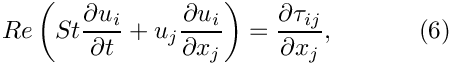with the global test functions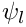, and integrating by parts to obtain the discrete residuals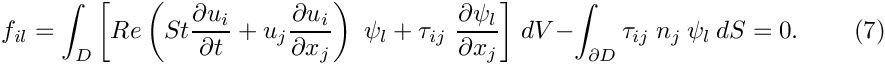The volume integral in this residual is computed by the "bulk" Navier-Stokes elements. Recall that in the residual for the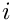-th momentum equation, the global test functionsvanish on those parts of the domain boundary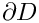where the-th velocity component is prescribed by Dirichlet boundary conditions. On such boundaries, the surface integral in (7) vanishes because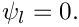If the velocity on a certain part of the domain boundary,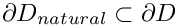, is not prescribed by Dirichlet boundary conditions and the surface integral over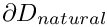is not added to the discrete residual, the velocity degrees of freedom on those boundaries are regarded as unknowns and the "traction-free" (or natural) boundary condition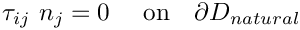is "implied". Finally, traction boundary conditions of the form (1) may be applied along a part,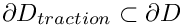, of the domain boundary. The surface integral along this part of the domain boundary is given by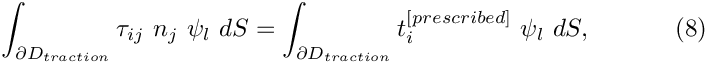where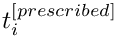is given, and it is this contribution that the traction elements add to the residual of the momentum equations.

## Exercises

1. Pin the vertical velocity along the upper boundary,, and compare the results against those obtained with the original version of the code. How does the change affect the velocity field? Why is pressure likely to change?
2. Pin the horizontal velocity along the upper boundary,, and start the simulation with an impulsive start. Compare the results against those obtained with the original version of the code and explain your findings, referring to the theory provided in the section How do the traction elements work? .
3. Run the code with an impulsive start and confirm that it takes longer for the solution to approach the time-periodic solution than in the previous case where the flow was driven by the wall motion. [Here are some animations of the velocity fields obtained following an impulsive start, for a discretisation with Taylor-Hood elements and Crouzeix-Raviart elements.]
4. Investigate the effects of applying a non-zero value for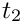on the top boundary.